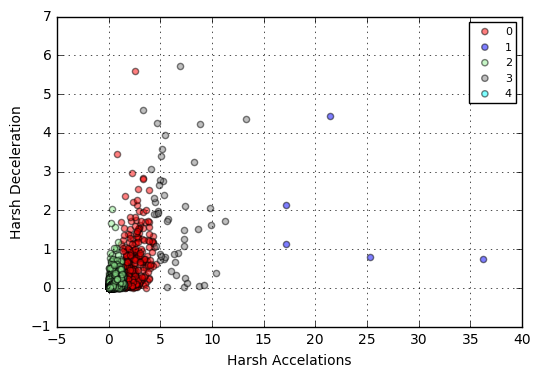# Massoud Mazar

## matplotlib charts in Jupyter notebook

When displaying graphs and charts in PySpark Jupyter notebook, you will have to jump through some hoops. To demonstrate, I'm assuming I have my K-Means clustering results as follows:

```model = KMeans(k=5, seed=1).fit(features.select('features'))
predictions = model.transform(features)```

You have to create a Temp View for this data, so you can run SQL on it:

`predictions.createOrReplaceTempView('cluster_predictions')`

And using SQL, you need to output the results using "-o":

```%%sql -q -o clusters
SELECT harshAcclRatio, harshDecelRatio, prediction FROM cluster_predictions```

Now, you need to run your plotting code locally:

```%%local
%matplotlib inline
import matplotlib.pyplot as plt

colors = ('red', 'blue', 'lightgreen', 'gray', 'cyan')

for i in range(0, 5):
data = clusters.where(clusters['prediction'] == i)
plt.scatter(data['harshAcclRatio'], data['harshDecelRatio'], alpha=0.5, label=str(i), c=colors[i])

plt.xlabel('Harsh Accelations')
plt.ylabel('Harsh Deceleration');
plt.legend(scatterpoints=1, fontsize=8)
plt.grid(True)
plt.show()```

and resulting scatter plot looks like this: